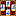###Rule presets

#### Chinese New Style

This rule preset represents the New Style version of the Chinese Mah Jong. This version of Mah Jong evolved originally among the leisure class in Peking and Shanghai (and is sometimes known as Shanghai Pai) and became popular in many parts of Asia (particularly in Taiwan) after the Second World War. The basic rules are the same as in other Chinese versions of Mah Jong, but here the emphasis is on collecting combinations in certain patterns (e.g., three similar Chows of different suits, three Pungs in sequence, etc.).

##### While there are dozens of different patterns, usually only some of them are selected for any one session of the game. In Four Winds a collection 18 new (non-classical) patterns have been specified for the rule preset, giving balanced rules suitable for players new to New Style, with enough patterns for variety without getting overwhelming. You can try with different patterns and save your favorites as user-defined presets.
 Tiles 136 (Flowers and Seasons are used occasionally; sometimes jokers, 4, 8 or 16, are used, as well; Four Winds allows a maximum of 8 jokers) Initial points 5,000 Limit points 64;  the maximum final score determined by the settling table is 4 laaks (64 points doubled four times, i.e., 1,024 points) earned by hands worth 20 faan or more. Restrictions for the winning hand None Claiming rules for going out Player first in turn wins Passing of the deal When non-dealer wins Rule of Ready None Dead hand rules None Payment To winner only; Chinese Modern scheme Discards In random order, no extended discard information Specialties Patterns emphasized; all Kongs accepted as concealed Pungs Scoring Faan-Laak system Basic tile points None Scores for basic sets Standard, but faan instead of doubles Scores for Flowers and Seasons Not used Scores for patterns based on Chows Same Chow from all suits (3 faan), Two identical Chows (2 faan), Two identical Chows twice (9 faan), 123 and 789 of the same suit (1 faan),  3 Chows of 1 suit, step 3 (3 faan) Scores for patterns based on Pungs and Kongs Triplets 1 and 9 of the same suit (1 faan), Little Three Dragons (6 faan) Scores for patterns based on the whole hand Exposed hand (all Kongs allowed, 2 faan), Concealed hand  (all Kongs allowed, 1 faan), Fully concealed hand (no Kongs allowed, 3 faan), Chow hand (no traditional value pairs allowed; 1 faan), Pung hand (3 faan), All Simples (1 faan), Terminal or Honor in each set (1 faan), Terminal in each set (2 faan), All Terminals and Honors (9 faan, Pung hand implied), All suits, Dragons and Winds (3 faan), Two suits only (2 faan), One Suit with Honors (3 faan), One Suit only (9 faan) Scores for miscellaneous patterns Four same in two sets (1 faan), Four same in three sets (1 faan), Four same in four sets (1 faan) Scores for winning Self-drawn last tile (1 faan), Out on a one-chance Chow (1 faan), Out on a pair (1 faan), Out on 4th of its kind (1 faan), Out on the last tile of the Wall (1 faan), Out on the last discard (1 faan), Out on a supplement tile (1 faan), Out by robbing a Kong (1 faan) Rounding None Penalties Faulty out: The deal continues normally but the offender pays 100% of Limit points (i.e., 64 points) to each of the other players, East does not pay/receive double; Faulty claims: None; Insurance penalties: Last five tiles error; Nine tiles error Limit hands Classical: Four Kongs (1600% of Limit), Hidden Treasure (1600%), Three Great Scholars (9 faan + components), Little Four Winds (12 faan + components), Big Four Winds (1600%), All Honors (1600%), All Terminals (1600%), Nine Gates (1600%) and Thirteen Orphans (1600%); Serpents: None; Pairs: None; Special ways of going out: Heavenly hand (1600%), Earthly hand (only from East's discard, 1600%), Kong on Kong (6 faan + components); American hands: None; Miscellaneous: None.
##### The final scores are determined by using a simple settling table where a certain amount of faan corresponds certain amount of final points. The following table shows the final payments per loser (notice that all pay double on a self-drawn winning tile, otherwise only the discarder pays double).

1 2+2+4=8 4+4+4=12
2 4+4+8=16 8+8+8=24
3 8+8+16=32 16+16+16=48
4 16+16+32=64 32+32+32=96
5 32+32+64=128 64+64+64=192
6 (1 laak) 64+64+128=256 128+128+128=384
7 74+74+148=296 148+148+148=444
8 84+84+168=336 168+168+168=504
9  (2 laaks) 128+128+256=512 256+256+256=768
10 138+138+276=552 276+276+276=828
11 148+148+296=592 296+296+296=888
12  (3 laaks) 256+256+512=1024 512+512+512=1536
13 266+266+532=1064 532+532+532=1596
14 276+276+552=1104 552+552+552=1656
15 (4 laaks) 512+512+1024=2048 1024+1024+1024=3072
16 522+522+1044=2088 1044+1044+1044=3132
17 532+532+1064=2128 1064+1064+1064=3192
18 542+542+1084=2168 1084+1084+1084=3252
19 552+552+1104=2208 1104+1104+1104=3312
20 (5 laaks) 1024+1024+2048=4096 2048+2048+2048=6144